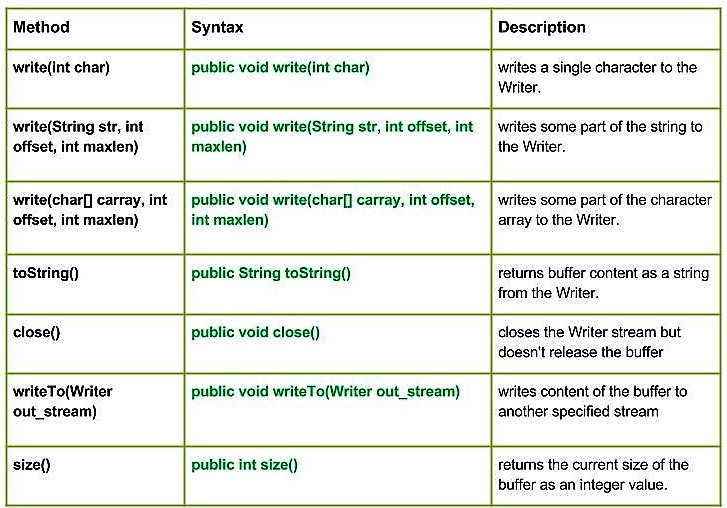Related Articles
Java.io.CharArrayWriter class in Java | Set 1
• Last Updated : 17 Jan, 2017java.io.CharArrayWriter class creates a character buffer that can be used as a writer. The buffer automatically grows when data is written to the stream. The data can be retrieved using toCharArray() and toString().
Declaration:

```public class CharArrayWriter
extends Writer```

Constructor :

• CharArrayWriter() : Creating a CharArrayWriter from a specified character array.
• CharArrayWriter(int size) : Creating a CharArrayWriter with the specified initial size.

Methods:

• write(int char) : java.io.CharArrayWriter.write(int char) writes a single character to the Writer.
Syntax:

```public void write(int char)
Parameters :
char : int value of the character to be written.
Return  :
void```
• write(String str, int offset, int maxlen) : java.io.CharArrayWriter.write(String str, int offset, int maxlen) writes some part of the string to the Writer.
Syntax:
```public void write(String str, int offset, int maxlen)
Parameters :
str : string to be written to the Writer.
offset : start position of the String
maxlen : maximum length upto which string has to written
Return  :
void```
• write(char[] carray, int offset, int maxlen) : java.io.CharArrayWriter.write(char[] carray, int offset, int maxlen) writes some part of the character array to the Writer.
Syntax:
```public void write(char[] carray, int offset, int maxlen)
Parameters :
carray : character to be written to the Writer
offset : start position of the character array
maxlen : maximum no. of the character of the carray has to written
Return  :
void```
• writeTo(Writer out_stream) : java.io.CharArrayWriter.writeTo(Writer out_stream) writes content of the buffer to another specified stream.
Syntax :
```public void writeTo(Writer out_stream)
Parameters :
out_stream : destination stream to be write into
Return  :
void
Exception :
IOException : In case of I/O error occurs```
• toString() : java.io.CharArrayWriter.toString() returns buffer content as a string from the Writer.
Syntax:
```public String toString()
Parameters :
-----------
Return  :
returns buffer content as a string from the Writer.```
• close() : java.io.StringWriter.close() closes the Writer stream but doesn’t release the buffer
Syntax:
```public void close()
Parameters :
-----------
Return  :
void```
• size() : java.io.StringWriter.size() returns the current size of the buffer as an integer value.
Syntax:
```public int size()
Parameters :
-----------
Return  :
integer value representing the current size of the buffer.```

Java code explaining use of CharArrayWriter class methods

 `// Java program illustrating the working of CharArrayWriter class methods``// write(int char), toString(), write(char[] carray, int offset, int maxlen)``// write(String str, int offset, int maxlen), size()`` ` `import` `java.io.*;``public` `class` `NewClass``{``    ``public` `static` `void` `main(String[] args) ``throws` `IOException``    ``{`` ` `        ``// Initailizing the character array``        ``char``[] geek = {``'G'``, ``'E'``, ``'E'``, ``'K'``, ``'S'``};``        ``String geek_str;`` ` `        ``// Initailizing the CharArrayWriter``        ``CharArrayWriter char_array1 = ``new` `CharArrayWriter();``        ``CharArrayWriter char_array2 = ``new` `CharArrayWriter();``        ``CharArrayWriter char_array3 = ``new` `CharArrayWriter();`` ` `        ``for``(``int` `c = ``72``; c < ``77``; c++)``        ``{``            ``// Use of write(int char)``            ``// Writer int value to the Writer``            ``char_array1.write(c);``        ``}`` ` `        ``// Use of toString() : returning Buffer content as String``        ``geek_str = char_array1.toString();``        ``System.out.println(``"Using write(int char) : "``+ geek_str);`` ` ` ` `        ``// Use of write(String str, int offset, int maxlen)``        ``// writes some part of the string to the Writer.``        ``char_array2.write(geek_str, ``2``, ``3``);`` ` `        ``System.out.println(``"write(str, offset, maxlen) : "``+ char_array2.toString());`` ` ` ` `        ``// Use of write(char[] carray, int offset, int maxlen)``        ``// writes some part of the Char[] geek to the Writer``        ``char_array3.write(geek, ``2``, ``3``);``        ``System.out.println(``"write(carray, offset, maxlen) : "``+ char_array3.toString());`` ` `        ``// get buffered content as string``        ``String str = char_array3.toString();`` ` ` ` `        ``// Use of writeTo(Writer out_stream)``        ``char_array3.writeTo(char_array1);`` ` `        ``System.out.println(``"\nchar_array3 to char_array1 : "``+ char_array1.toString());`` ` ` ` `        ``// Use of size() method``        ``System.out.println(``"\nSize of char_array1 : "``+ char_array1.size());``        ``System.out.println(``"Size of char_array1 : "``+ char_array2.size());``        ``System.out.println(``"Size of char_array1 : "``+ char_array3.size());`` ` `    ``}``}`

Output :

```Using write(int char) : HIJKL
write(str, offset, maxlen) : JKL
write(carray, offset, maxlen) : EKS

char_array3 to char_array1 : HIJKLEKS

Size of char_array1 : 8
Size of char_array1 : 3
Size of char_array1 : 3```

Next Article: Java.io.CharArrayWriter class in Java | Set2

This article is contributed by Mohit Gupta 🙂. If you like GeeksforGeeks and would like to contribute, you can also write an article using contribute.geeksforgeeks.org or mail your article to contribute@geeksforgeeks.org. See your article appearing on the GeeksforGeeks main page and help other Geeks.